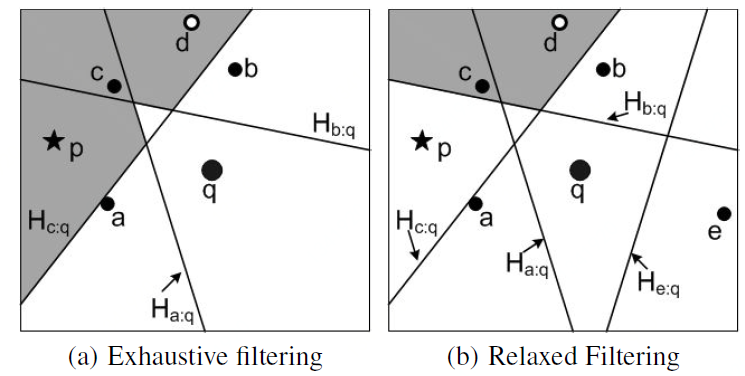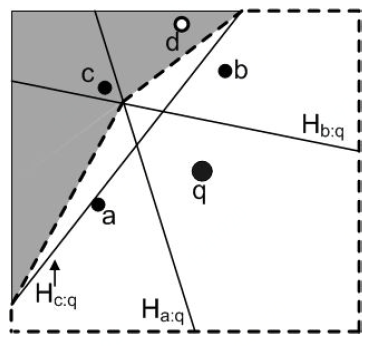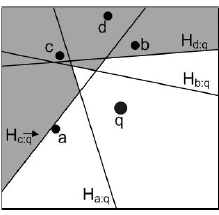# 多维反向k近邻查询

Published:

[本文总阅读量: 次]

JUST技术：多维反向k近邻查询
JUST-团队 何华均

## 基本定义和步骤

R$k$NN查询分为双色R$k$NN查询和单色R$k$NN查询。

Filtering: 过滤阶段，每个算法使用一组设施来过滤不包含R$k$NN的搜索空间。由于使用所有数据的可能非常昂贵，算法首先会挑选一些设施来过滤搜索空间。这些设施称为过滤设施，包含这些设施的集合称为过滤集(表示为$S_{fil}$)。

**Verification: **验证阶段，检索不能使用$S_{fil}$过滤的用户，它们是可能的R$k$NN，称为候选用户。然后通过确认它是否是R$k$NN来验证每个候选用户。

## 算法

(1) Six-regions

Filtering: Stanoi等人提出了一种基于Six-regions(6个区域)的方法，将以查询q为中心的整个空间划分为6个相等的区域，每个区域60$^{\circ}$，(下图中的P1到P6)。每个区域中，q的第k个最接近的设施定义了可以过滤的区域。换句话说，假设$d_i^k$是区域$P_i$中q与其第k个最近的设施之间的距离。那么任何在$P_i$中并且距离q大于$d_i^k$的用户u都不能是q的R$k$NN。Verification: 如前所述，位于分区$P_i$中的用户u，如果$dist(u,q) > d_i^k$，则$u$不能是$q$的R$k$NN 。在验证阶段，通过访问用户R*-tree来检索候选用户，并过滤mindist(e, q) >$d_i^k$的节点, 并通过Filtering步骤过滤$dist(u,q) > d_i^k$的用户，得到候选用户。然后每个候选用户通过一个布尔范围query来验证是否是q的R$k$NN。布尔查询以u为中心，半径为$dist(u, q)$作圆。当且仅当在以u为中心的圆中只有小于k个设施时，返回true，$u$是$q$的R$k$NN。如图(b)所示，以$u$为圆心，半径为$dist(u,q)$的圆，只包含两个设施。因此$u$为$q$的R$k$NN(k=2)。

(2) TPL

Filtering: Tao等人[4，5]提出的TPL可以说是R$k$NN查询中最流行的算法。他们首次将半空间剪枝的概念用于R$k$NN查询，并激发了许多后续工作。给定一个设施f和一个查询q, fq之间的一条垂直平分线$B_{f:q}$ 将空间分成两半。设$H_{f:q}$表示包含f的半空间，$H_{q:f}$表示包含q的半空间，位于$H_{f:q}$中的每个点p，都满足$dist(p, f) < dist(p, q)$，也就是说，f可以裁剪位于$H_{f:q}$中的每个点pTPL的过滤算法从根节点开始访问设施R*-tree的节点，并将它们按与q的最小距离升序排列放入堆中。迭代地访问堆，如果一个被访问的实体e（可以是一个节点或者一个设施）可以被过滤(例如，e被至少k个设施裁剪)，它将被忽略。否则，如果e是中间节点或叶节点，则将其子节点插入堆中。另一方面，如果e是一个设施，且无法裁剪，则将其插入过滤集$S_{fil}$中，其半空间用于过滤搜索空间。当堆为空时，过滤算法终止。在图(a)中，假设过滤算法以$b、c、a、d$的顺序迭代访问设施，当访问设施$b、c、a$时(即$S_{fil}= {b, c, a}$)，过滤区域由半空间$H_{b:q}, H_{c:q}, H_{a:q}$的交集组成。如果k = 2，则阴影区域可以被过滤，因为其中的每个点都位于至少两个半空间中。如，当访问设施d时，可以使用筛选集对它进行剪枝。因此，在$S_{fil}$中不用插入d

Verification： TPL迭代地访问用户R*-tree的节点，并使用$S_{fil}$对它们进行过滤。无法筛选的用户被插入到候选集中。与六个区域不同，TPL不会使用布尔范围查询来验证候选区域。取而代之地，TPL使用了一种更聪明的策略，它要求R*-tree的每个节点最多访问一次。具体地说，在过滤阶段遇到的设施R*-tree的节点和数据点保持在集合P中，验证算法轮询运行。在每一轮中，P中的一个节点被取出，并将其子节点被插入到P中。选择被取出的节点是基于它可以验证多少个候选节点。在每一轮中，利用P中的节点和数据点来识别可以用P验证的候选项，即可以确认为R$k$NN或保证不为R$k$NN。这样的候选项将被验证并从候选者集中删除。当候选集为空时，算法停止。

(3) FINCH

Filtering: Wu等人提出了一种名为FINCH的算法，旨在通过使用一种更方便的过滤策略来改进TPL。具体来说，它不使用子集来过滤节点，而是使用一个包围不能被过滤区域的凸多边形。多边形之外的任何条目都可以被过滤。考虑图(a)的例子，其中白色区域是非过滤的区域。FINCH用一个凸多边形来近似这个区域(参见下图中的白色区域，边界用虚线表示)。任何在这个多边形之外的节点都可以被过滤，也就是阴影部分。

Verification: 识别凸多边形内的用户并将其插入到候选集中。与6区域方法类似，每个候选用户都需要使用布尔范围查询进行验证。(4) InfZone

Filtering: Cheema等人[1，2]提出InfZone算法，利用影响区域的概念来改善验证阶段。影响区域是指当且仅当p位于该区域内时，点pq的R$k$NN的区域。一旦计算了影响区域，就可以通过定位位于影响区域内的用户来回答R$k$NN查询。一种方法建立影响区的方法是绘制所有设施的半空间，被小于k个设施过滤的区域对应于影响区。例如，在下图中，q和所有设施之间的半空间被绘制为$Ha:q, Hb:q, Hc:q，和Hd:q$, 则阴影区域是过滤区域，白色区域是影响区域。回想一下，FINCH和TPL没有考虑$Hd:q$，因为当访问d时，会发现它位于过滤区域，因此会被忽略。作者提出了几个性质，以减少必须考虑的设施的数量，以正确计算合适的影响区域。该算法首先初始化影响区域为整个数据空间(矩形)。每当访问设施f时，其半空间$Hf:q$用于更新影响区，例如，通过删除至少k个设施修剪的部分。对于当前影响区域的每个顶点v，如果$minDist(e,v) > dist(v,q)$, 则不需要这个实体e(一个节点或一个设施)计算影响区域。因此，该算法只考虑不满足该条件的实体。请注意，检查是否需要设施实体需要$O(m)$，因为遍历影响区域的顶点数为$O(m)$。当在$S_{fil}$中插入新设施时，更新影响区域需要$O(m^2)$，其中m是$S_{fil}$中的设施总数。在它的扩展版中，该成本优化到了$O(km)$。Verification: 根据影响区域的定义，当且仅当p在影响区域内时，点p为R$k$NN。因此，算法将迭代访问用户R*-tree的实体，并忽略不与影响区域重叠的实体。位于影响区域内的用户则为R$k$NN。结果表明，影响区域为星形多边形，点包含在星形多边形边数可以在对数时间内完成，即验证用户的成本为$O(log~ m)$。为了过滤一个用户节点(即一个矩形)，需要判断矩形是否与影响区相交，它花费$O(m)$。为了加快检查某个实体(节点或点)是否与影响区域重叠，InfZone被近似为两个圆:一个完全包含影响区域，另一个完全包含它（所有影响区域）。首先对照这些圆检查每个实体的重叠部分，以确定是否与影响区重叠。

## 参考文献：

 M. A. Cheema, X. Lin, W. Zhang, and Y. Zhang. Influence zone: Efficiently processing reverse k nearest neighbors queries. In ICDE, pages 577–588, 2011.

 M. A. Cheema, W. Zhang, X. Lin, and Y. Zhang. Efficiently processing snapshot and continuous reverse k nearest neighbors queries. VLDB J., 21(5):703–728, 2012.

 I. Stanoi, D. Agrawal, and A. E. Abbadi. Reverse nearest neighbor queries for dynamic databases. In ACM SIGMOD Workshop, pages 44–53, 2000.

 Y. Tao, D. Papadias, and X. Lian. Reverse knn search in arbitrary dimensionality. PVLDB, pages 744–755, 2004.

 Y. Tao, D. Papadias, X. Lian, and X. Xiao. Multidimensional reverse k nn search. VLDB J., 16(3):293–316, 2007.

 W. Wu, F. Yang, C. Y. Chan, and K.-L. Tan. Finch: Evaluating reverse k-nearest-neighbor queries on location data. PVLDB, 1(1):1056–1067, 2008.

 Yang, S., Cheema, M. A., Lin, X., & Wang, W. (2015). Reverse k nearest neighbors query processing: experiments and analysis. PVLDB, 8(5), 605-616.

Tags: